ELECTRONIC STRUCTURE AND ATOMIC ORBITALS

```
```

A simple view

In any introductory chemistry course you will have come across the electronic structures of hydrogen and carbon drawn as: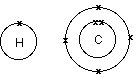Note:  There are many places where you could still make use of this model of the atom at A' level. It is, however, a simplification and can be misleading. It gives the impression that the electrons are circling the nucleus in orbits like planets around the sun. As you will see in a moment, it is impossible to know exactly how they are actually moving.

The circles show energy levels - representing increasing distances from the nucleus. You could straighten the circles out and draw the electronic structure as a simple energy diagram.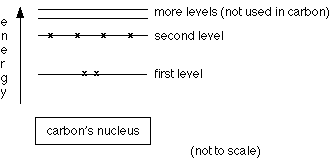Atomic orbitals

Orbits and orbitals sound similar, but they have quite different meanings. It is essential that you understand the difference between them.

The impossibility of drawing orbits for electrons

To plot a path for something you need to know exactly where the object is and be able to work out exactly where it's going to be an instant later. You can't do this for electrons.

Note:  In order to plot a plane's course, it is no use knowing its exact location in mid-Atlantic if you don't know its direction or speed. Equally it's no use knowing that it is travelling at 500 mph due west if you have no idea whether it is near Iceland or the Azores at that particular moment.

The Heisenberg Uncertainty Principle (not required at A'level) says - loosely - that you can't know with certainty both where an electron is and where it's going next. That makes it impossible to plot an orbit for an electron around a nucleus. Is this a big problem? No. If something is impossible, you have to accept it and find a way around it.

Hydrogen's electron - the 1s orbital

Note:  In this diagram (and the orbital diagrams that follow), the nucleus is shown very much larger than it really is. This is just for clarity.Suppose you had a single hydrogen atom and at a particular instant plotted the position of the one electron. Soon afterwards, you do the same thing, and find that it is in a new position. You have no idea how it got from the first place to the second.

You keep on doing this over and over again, and gradually build up a sort of 3D map of the places that the electron is likely to be found.

In the hydrogen case, the electron can be found anywhere within a spherical space surrounding the nucleus. The diagram shows a cross-section through this spherical space.

95% of the time (or any other percentage you choose), the electron will be found within a fairly easily defined region of space quite close to the nucleus. Such a region of space is called an orbital. You can think of an orbital as being the region of space in which the electron lives.

Note:  If you wanted to be absolutely 100% sure of where the electron is, you would have to draw an orbital the size of the Universe!

What is the electron doing in the orbital? We don't know, we can't know, and so we just ignore the problem! All you can say is that if an electron is in a particular orbital it will have a particular definable energy.

Each orbital has a name.

The orbital occupied by the hydrogen electron is called a 1s orbital. The "1" represents the fact that the orbital is in the energy level closest to the nucleus. The "s" tells you about the shape of the orbital. s orbitals are spherically symmetric around the nucleus - in each case, like a hollow ball made of rather chunky material with the nucleus at its centre.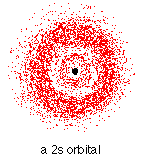The orbital on the left is a 2s orbital. This is similar to a 1s orbital except that the region where there is the greatest chance of finding the electron is further from the nucleus - this is an orbital at the second energy level.

If you look carefully, you will notice that there is another region of slightly higher electron density (where the dots are thicker) nearer the nucleus. ("Electron density" is another way of talking about how likely you are to find an electron at a particular place.)

2s (and 3s, 4s, etc) electrons spend some of their time closer to the nucleus than you might expect. The effect of this is to slightly reduce the energy of electrons in s orbitals. The nearer the nucleus the electrons get, the lower their energy.

3s, 4s (etc) orbitals get progressively further from the nucleus.

p orbitalsNot all electrons inhabit s orbitals (in fact, very few electrons live in s orbitals). At the first energy level, the only orbital available to electrons is the 1s orbital, but at the second level, as well as a 2s orbital, there are also orbitals called 2p orbitals.

A p orbital is rather like 2 identical balloons tied together at the nucleus. The diagram on the right is a cross-section through that 3-dimensional region of space. Once again, the orbital shows where there is a 95% chance of finding a particular electron.

Beyond A'level:   If you imagine a horizontal plane through the nucleus, with one lobe of the orbital above the plane and the other beneath it, there is a zero probability of finding the electron on that plane. So how does the electron get from one lobe to the other if it can never pass through the plane of the nucleus? For A'level chemistry you just have to accept that it does! If you want to find out more, read about the wave nature of electrons.

Unlike an s orbital, a p orbital points in a particular direction - the one drawn points up and down the page.

At any one energy level it is possible to have three absolutely equivalent p orbitals pointing mutually at right angles to each other. These are arbitrarily given the symbols px, py and pz. This is simply for convenience - what you might think of as the x, y or z direction changes constantly as the atom tumbles in space.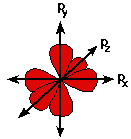The p orbitals at the second energy level are called 2px, 2py and 2pz. There are similar orbitals at subsequent levels - 3px, 3py, 3pz, 4px, 4py, 4pz and so on.

All levels except for the first level have p orbitals. At the higher levels the lobes get more elongated, with the most likely place to find the electron more distant from the nucleus.

Fitting electrons into orbitals

Because for the moment we are only interested in the electronic structures of hydrogen and carbon, we don't need to concern ourselves with what happens beyond the second energy level.

Remember:

At the first level there is only one orbital - the 1s orbital.

At the second level there are four orbitals - the 2s, 2px, 2py and 2pz orbitals.

Each orbital can hold either 1 or 2 electrons, but no more.

"Electrons-in-boxes"

Orbitals can be represented as boxes with the electrons in them shown as arrows. Often an up-arrow and a down-arrow are used to show that the electrons are in some way different.

Beyond A'level:  The need to have all electrons in an atom different comes out of quantum theory. If they live in different orbitals, that's fine - but if they are both in the same orbital there has to be some subtle distinction between them. Quantum theory allocates them a property known as "spin" - which is what the arrows are intended to suggest.A 1s orbital holding 2 electrons would be drawn as shown on the right, but it can be written even more quickly as 1s2. This is read as "one s two" - not as "one s squared".

You mustn't confuse the two numbers in this notation:The order of filling orbitals

Electrons fill low energy orbitals (closer to the nucleus) before they fill higher energy ones. Where there is a choice between orbitals of equal energy, they fill the orbitals singly as far as possible.

The diagram (not to scale) summarises the energies of the various orbitals in the first and second levels.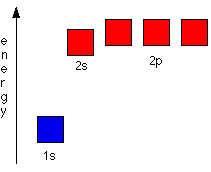Notice that the 2s orbital has a slightly lower energy than the 2p orbitals. That means that the 2s orbital will fill with electrons before the 2p orbitals. All the 2p orbitals have exactly the same energy.

The electronic structure of hydrogen

Hydrogen only has one electron and that will go into the orbital with the lowest energy - the 1s orbital.

Hydrogen has an electronic structure of 1s1. We have already described this orbital earlier.

The electronic structure of carbon

Carbon has six electrons. Two of them will be found in the 1s orbital close to the nucleus. The next two will go into the 2s orbital. The remaining ones will be in two separate 2p orbitals. This is because the p orbitals all have the same energy and the electrons prefer to be on their own if that's the case.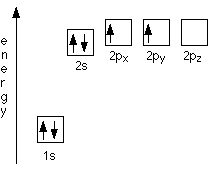Note:  People sometimes wonder why the electrons choose to go into the 2px and 2py orbitals rather than the 2pz. They don't! All of the 2p orbitals are exactly equivalent, and the names we give them are entirely arbitrary. It just looks tidier if we call the orbitals the electrons occupy the 2px and 2py.

The electronic structure of carbon is normally written 1s22s22px12py1.

```
```
 Questions to test your understanding If this is the first set of questions you have done, please read the introductory page before you start. You will need to use the BACK BUTTON on your browser to come back here afterwards. questions on orbitals answers
```
```

Where would you like to go now?

To the organic bonding menu. . .

To menu of basic organic chemistry. . .

To Main Menu . . .

```
```

© Jim Clark 2000 (modified February 2013)